• In the previous lesson, we learned about the USGS earthquake data and some of the limits of map visualizations.
• In this lesson, we use basic spreadsheet techniques to create slightly more basic, but more insightful visualizations.
• In the next lesson, we use pivot tables to summarize the data to add an essential aggregate layer to our bar chart visualizations.

# Import the data into a spreadsheet

Then import it into a Google Spreadsheet. You shouldn't have to change any of the default options. The delimiter, which of course is a comma for our data set, should be automatically detectable by Google's import process.

The result of the import should look something like this, i.e. a typical spreadsheet: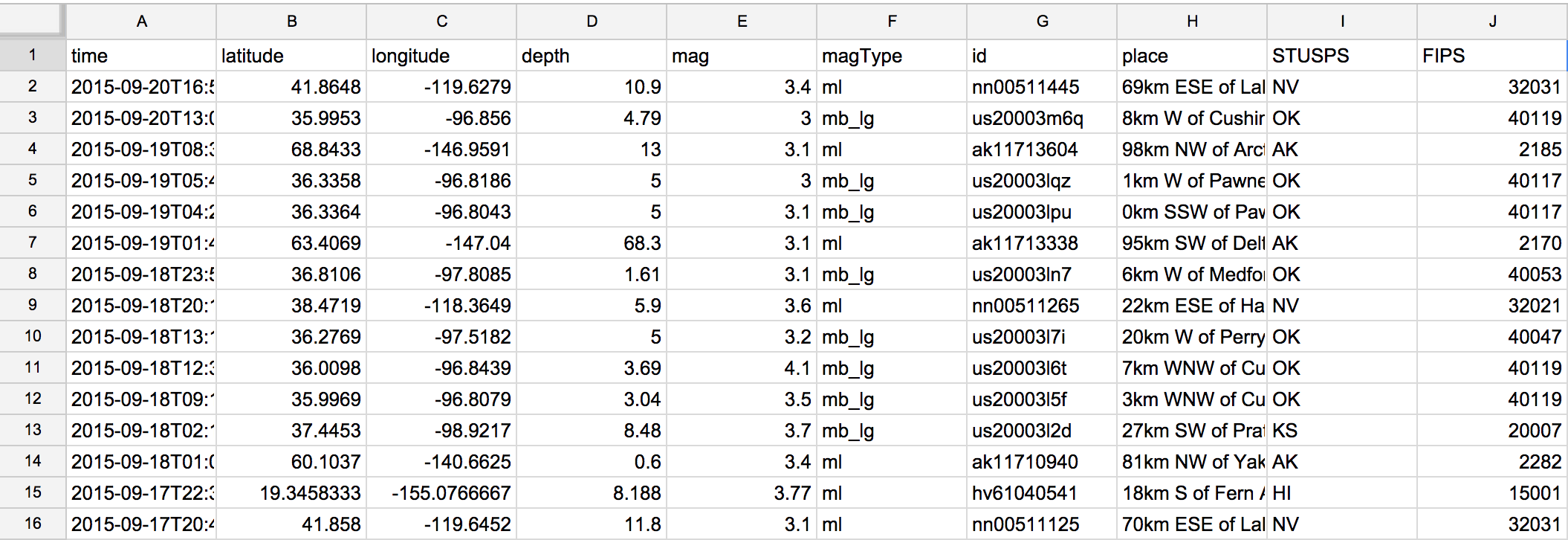Creating a chart from a spreadsheet is a matter of selecting the data-to-be visualized. Then on the menubar, select Insert, then select the Chart… menu item.

Selecting the data is the part that requires thinking. For now, let's just highlight the mag column. In modern spreadsheets, the easiest way to highlight an entire column is to click the column's header.

In the animated GIF below, I demonstrate highlighting the data column and choosing the Insert > Chart… menu item: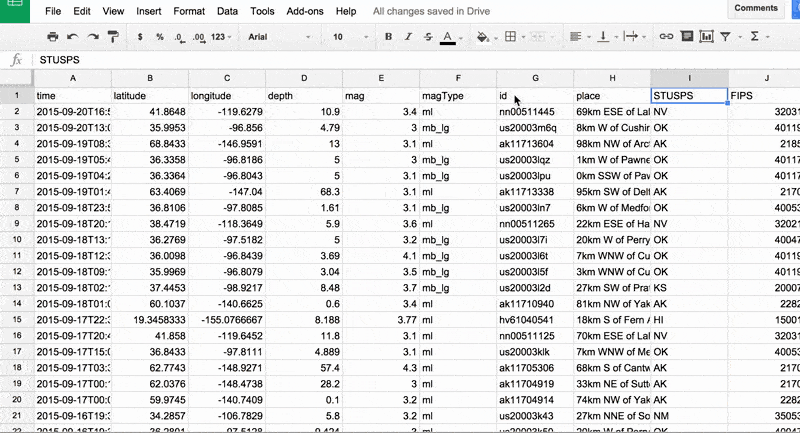### Bar chart of magnitudes

As soon as we try to create a chart, the first thing Google Sheets offers us is a selection of "Recommended" charts. How does it know what to recommend? Well, we don't know, which is why in general, we need to learn how to choose chart types for ourselves. But a good guess is that the Google Sheets program sees that our data selection is a single column of numbers and knows, by convention, that there are a few likely choices for visualization options.

That a computer can predict what chart type we might use should be a hint that the very structure of the data selection is critical in what type of visualizations can be created: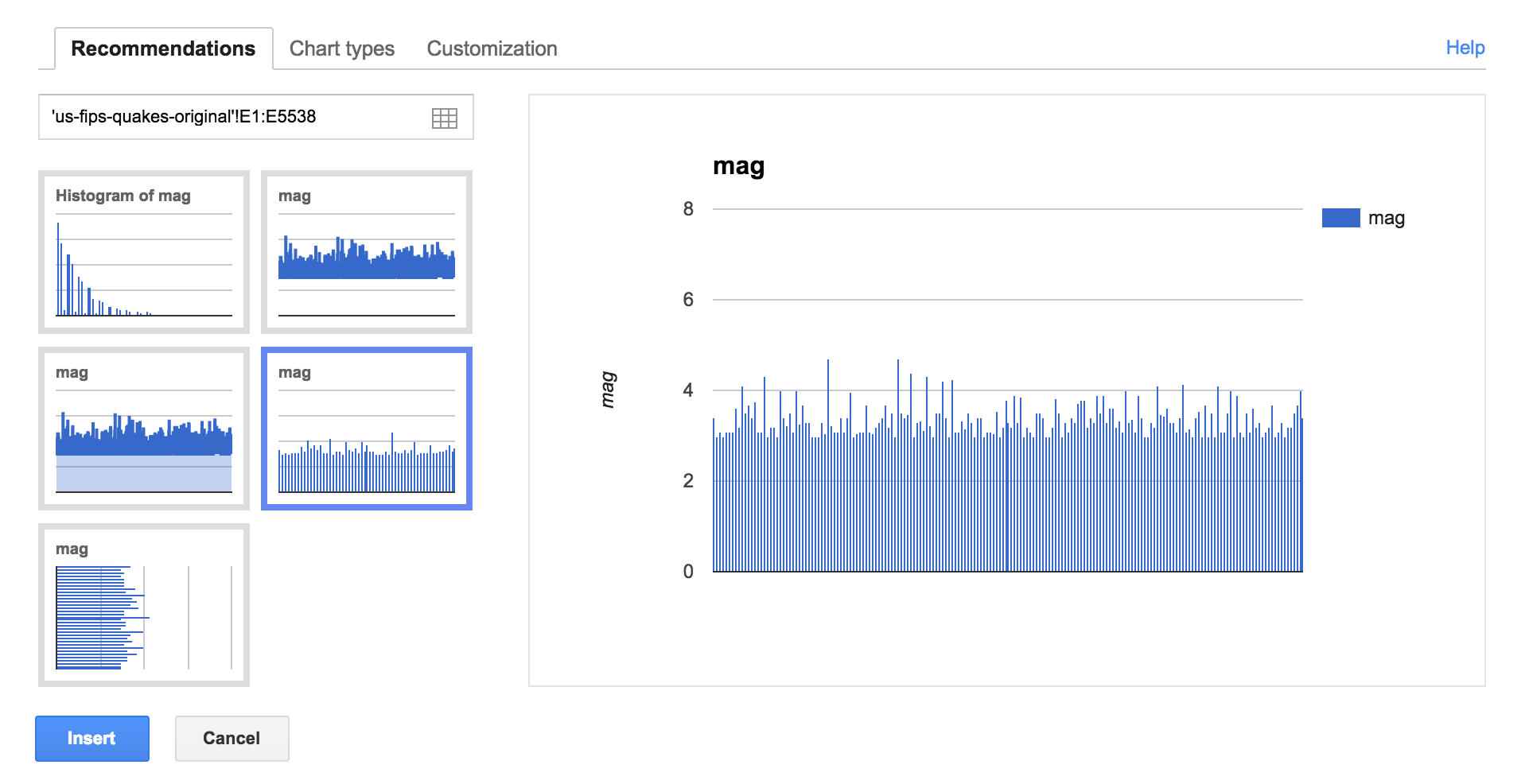The histogram is actually the best choice, but for now, I choose the one that seems to be a straightforward bar chart, in which each earthquake is represented by a very thin line. The height of the line (i.e. the y-axis) depicts the mag value of each earthquake:

It's actually not too bad. We can see with a quick glance that other than very few earthquakes break the 5.0 mark. So this is definitely a visual refinement of what we started out with: a bunch of text, in which reading all the mag values is very cumbersome. But that's about the limit of our insights.

### Scatterplot, longitude vs. latitude

Before moving on to the histogram-type chart, let's try charting a different part of the data. Highlight both the longitude and latitude columns, and then selecting Insert > Chart…

The very first recommended chart should be a scatter plot. As I mentioned in the previous lesson, a map can be thought of as a scatter plot with longitude and latitude on the x- and y-axis, respectively:

For whatever reason, though, I can't get Google to not switch those two things around. Oh well, you can mentally transpose the variables yourself, then imagine drawing the outlines of the United States onto the scatter plot. And you basically have a geospatial map. Moving back to magnitudes and histograms…

# Histograms

Think of a histogram as a special kind of bar chart that shows how the data points are distributed.

This is similar in purpose to the binned maps we saw in the previous lesson: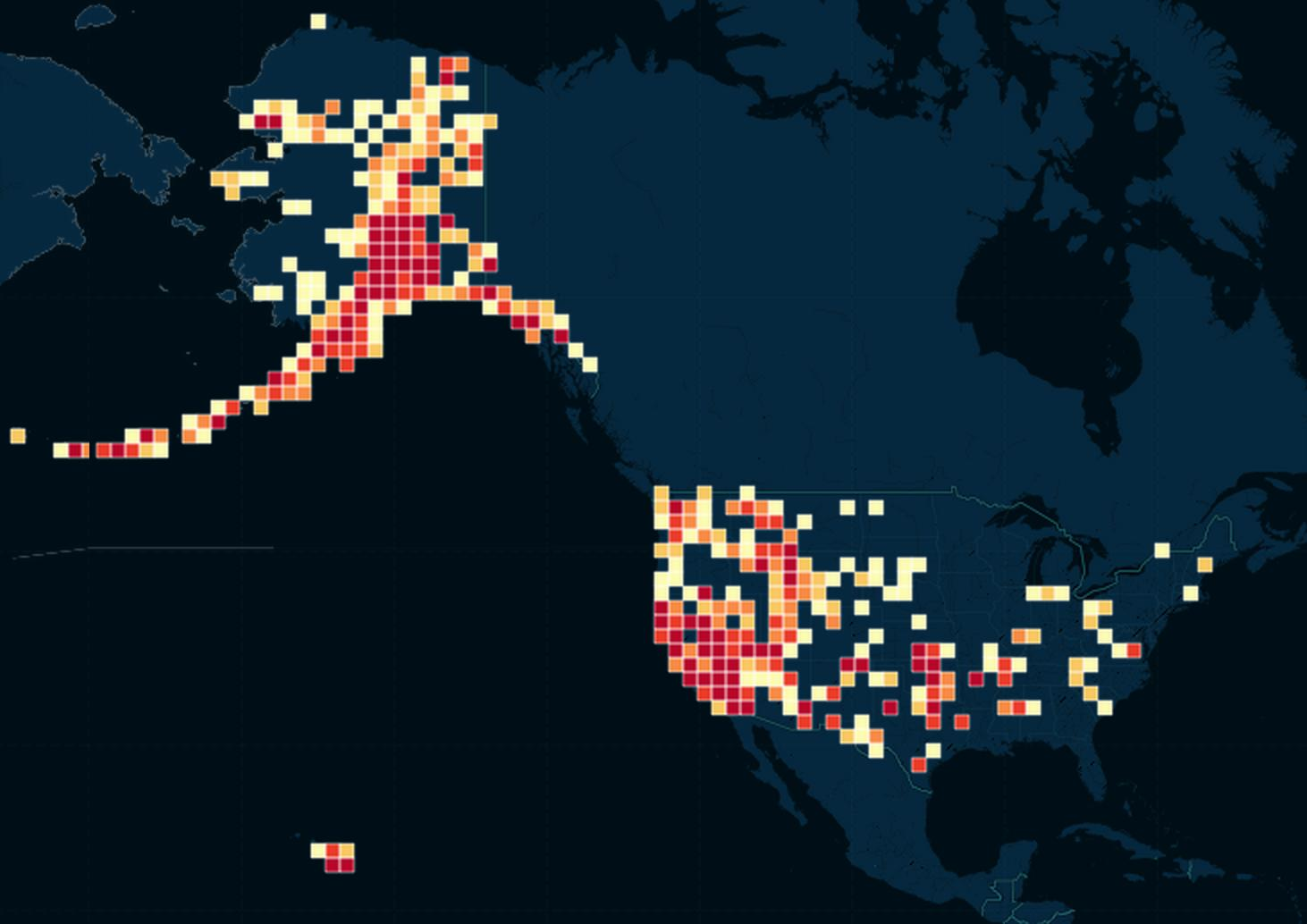Earthquakes similar in geospatial location were represented as a single bubble/square/hexagon, and the color of this clustered shape indicated how many earthquakes were in that cluster. In the example above, the redder the square, the more earthquakes in that area. This density map depicts the distribution of earthquakes along two dimensions – latitude and longitude – and color is used to show the amount (i.e. the density) of the distribution.

In contrast, a histogram depicts only one dimension and uses the height of the bar (i.e. the y-axis) to show the amount of each distribution.

## Histogram of magnitudes

Remember our first attempt at charting the mag column? We can see the outliers, but we can't easily see the distribution of the earthquakes by magnitude, e.g. how many earthquakes are M4.0+?, etc.

So highlight the mag column, select the menu action to Insert > Chart…, and then from the list of recommended chart types, select Histogram.

You should get something similar to this:

It's definitely a lot fewer bars to look at, and it tells the general story of the distribution: there are way more M4.0-or-weaker earthquakes. But it's a little awkward because of the bins, which you can see in the x-axis where the ticks are spaced un-neatly at intervals of 0.26.

It's is a bid weird how every third bar is a major drop-off from the first two. I can't tell if that's because the data itself is that weird, or just a quirk in the graphing process, in which some values are not rounded the way they should be.

Either way, the default bins that Google chose aren't great. So let's make our own.

# The importance of bucket size

I didn't go demonstrate this in the previous lesson, but it's not just the shape of the bins (e.g. hexagons versus squares), but the size of the bins that can be varied, and which can make a big difference in how the data is interpreted.

Below are three variations of sizes of the hexbins: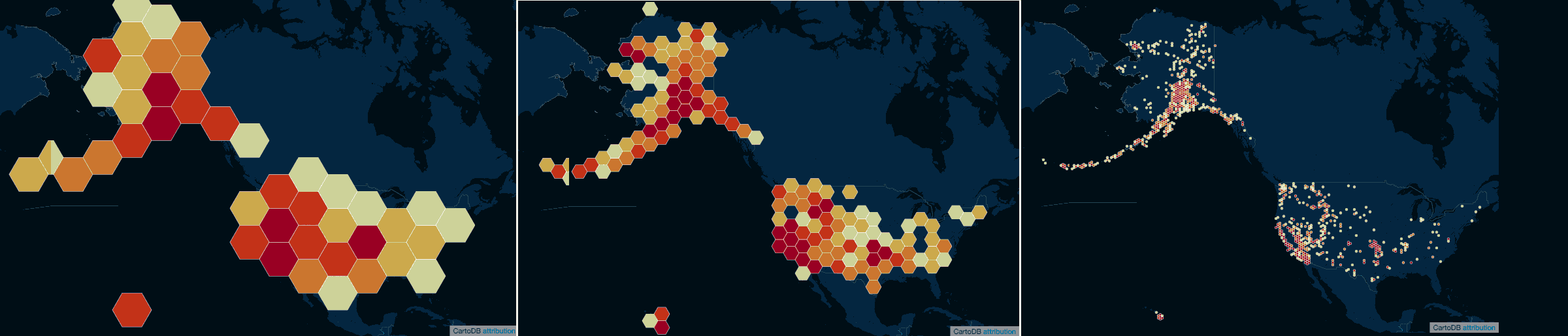If the bins – often referred to as buckets – are too big, then we lose valuable geographic distinctions: the bins for California earthquakes spill across the border and make the entire West coast a block of red. But make the buckets too small, and we're back right at the original problem: having too much data to visually interpret.

This same problem applies to histograms. In our first attempt, the default bucket size was too small…or just too weird. Either way, the visual story was jumbled.

## Magnitude bucketed by integers

So let's go for "big" bucket sizes for our histogram of magnitudes. Round all magnitude values to the nearest integer. This is better done explicitly, by making a new column and using a formula. But we get to formulas later, let's see what magic the Google charting software can do for us.

Repeat the part where you select the mag columns and perform an Insert Chart. When the recommended visualizations pop up, select the Histogram option again, but then, click on the Customization tab.

Then scroll down until you see the Buckets sub-menu. In the input field for Bucket size, enter a value of `1`: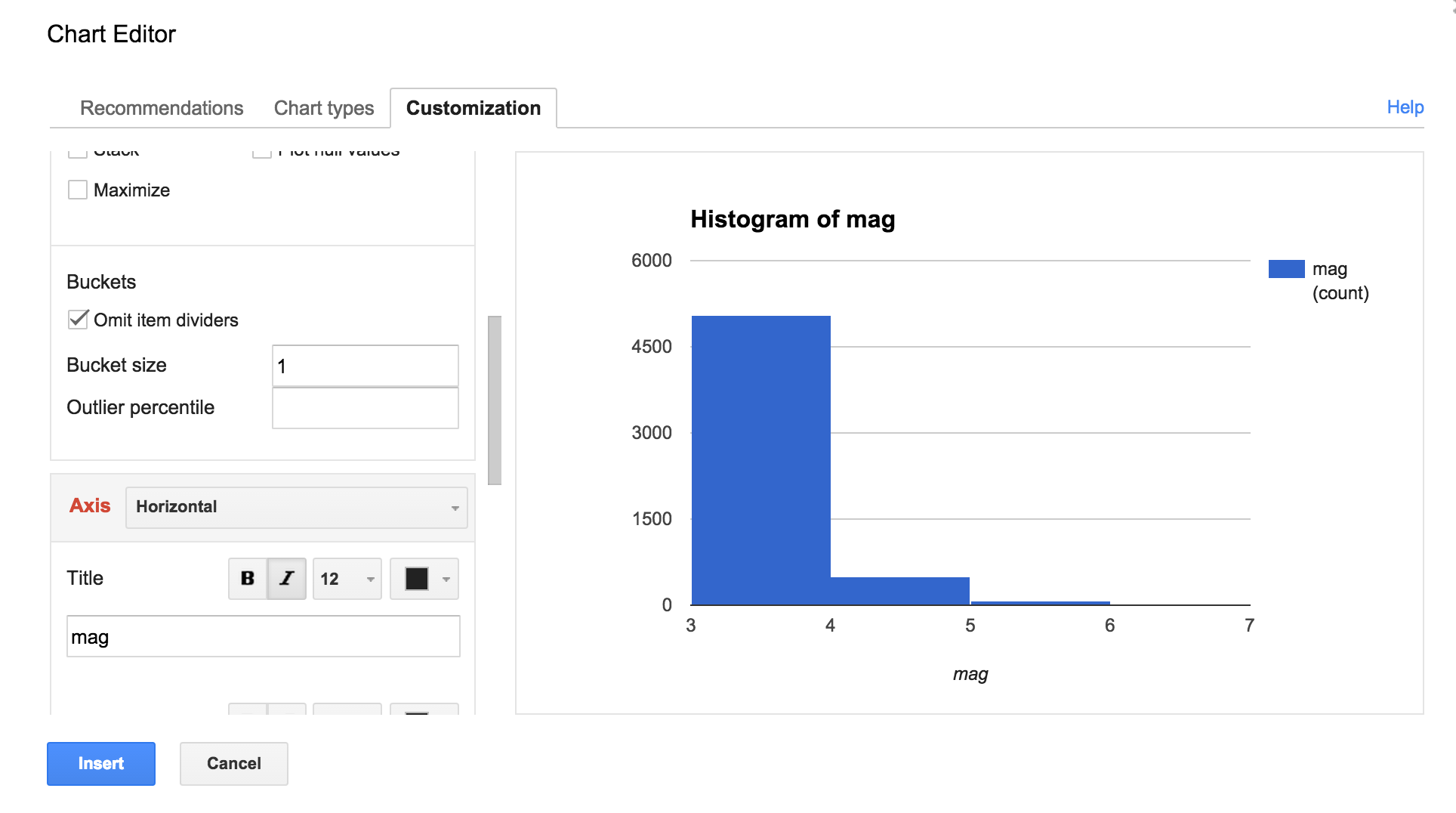This is the resulting chart:

As we guessed earlier, earthquakes M4.0-and-weaker make up the vast majority of earthquakes. And earthquakes M5.0+ and above were very rare.

### Magnitude bucketed by 0.2 increments

Let's customize the bucket size again and see what a smaller size looks like. This is especially appropriate with our given data set because, if you recall from geology class, the power of an earthquake is logarithmic, i.e. a M4.0 earthquake is 10 times the amplitude of a M3.0 earthquake.

So let's choose a fraction of a number for the bucket. Here's what a bucket size of 0.2 looks like:

Is this particularly profound? Maybe, if you care that there's a large drop-off after M3.4+. Otherwise, the story is not much different from the integer-bucketed histogram, at least to us laypersons.

# Categorical histograms

We can use histograms using non-numerical columns, such as the STUSPS columns, in which case we don't care about "bucket size". This kind of histogram shows a distribution by category.

## Histogram of states

In the case of STUSPS, the category is the name of the U.S. state the quake was centered in. Highlight the STUSPS column and repeat the steps to create a histogram:

This sorting is not optimal. And also, not all 50 states are fit into the chart or even labeled. This is a limitation of the charting software. Stupid Google you might think. But actually, stupid us for trying to graph all 50 states. Some are clearly not worth showing as they have so little earthquakes throughout their history.

One way to get around the limitations of the histogram is to use a pie chart. Except Edward Tufte thinks pie charts are bad. But maybe because he hasn't seen them in faux interactive 3-D:

If only we could limit the data to only the top 10-or-so states by total earthquake activity, we'd have a nice chart, pie or histogram. Unfortunately, I don't think there's an easy way to do kind of filtering with vanilla spreadsheets. But it's easy with Pivot Tables, which we will get to soon enough in the next lesson.

# Deriving new columns with formulas and functions

In the previous lesson, I came up with a lame attempt to show earthquakes-by-ear using the CartoDB map: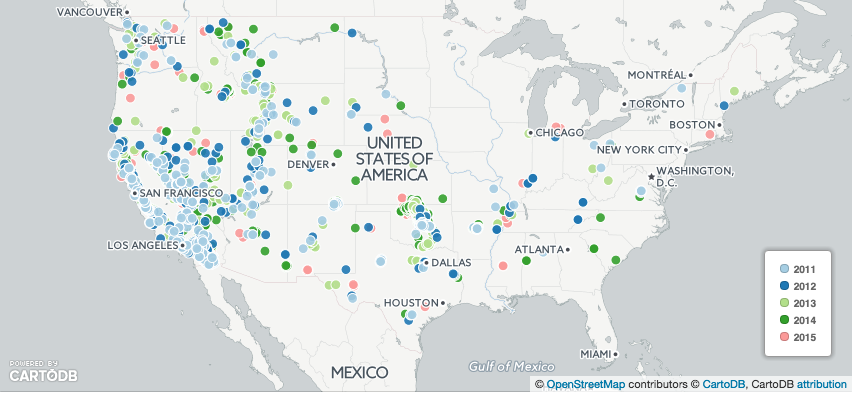So many of the earthquake points overlap that it's hard to tell if 2011 was a dominant year in earthquakes, or if the charting software just happened to place 2011 earthquakes on top of the later quakes by default.

So right now, we just want to figure out: how many earthquakes were there per year?

Unfortunately, while our dataset does have a time column, there is no column for just year. So let's make one.

## The `LEFT` function

There's several approaches we could take in order to derive the year from each time value. Just to warn you: working with time – or rather, the datatypes and values that purport to represent time – is one of those things that continues to be very frustrating for me, even as an experienced programmer.

Rather than jump into that pain, let's tackle the problem in the most literal, straightforward way.

Here is a sample value from the time column:

``````    2015-09-20T16:55:21.558Z
``````

What part of that text string represents the year? The first 4 characters, e.g. `2015`. So to create a year column, we just need to extract the first 4 characters of every value in the time column.

There is a function in Google Sheet that does exactly this: LEFT. Via Google's documentation:

Sample usage:

``````    LEFT(A2,2)
``````

In which `A2` is the reference to a cell, and `2` refers to number of characters to extract, starting from the left side.

## How to create and repeat a formula

At this point, I'm going to assume you kind of know what a spreadsheet formula is and how they work, generally. If not, check out Google's help page here.

Below is an animated GIF in which I create a year column. Note these things:

• The time column in our dataset is in the first column, i.e. column A
• Successfully running a formula requires that, in a given cell, you first enter an equals sign, i.e. `=LEFT(A2,4)`
• To repeat the formula down an entire column, first make sure it works once. Then click on the cell that you want to repeat to highlight it. Then double-click its bottom-right-corner – Google Sheet should make it obvious by depicting that corner with a blue square.

Any way, here's the GIF: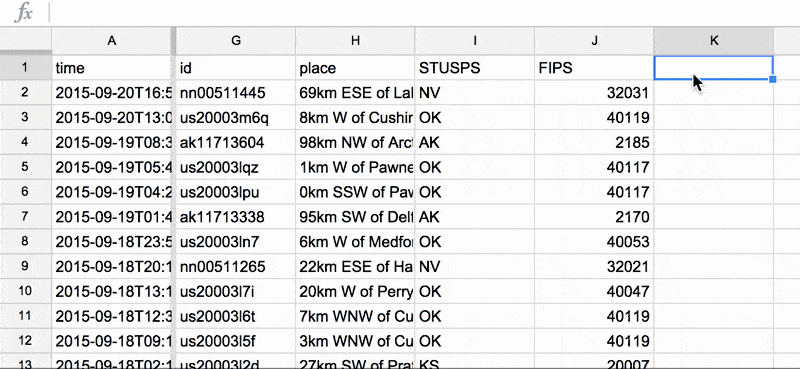### Histogram by year

Let's get back to chart-making. Highlight our new year column and create a time series – which is basically a histogram except having some kind of time value in the x-axis.

It should look something like this:

Note: I've adjusted a few of the chart editor options, such as removing the Legend and title for the y-axis. I'm going to be doing that for all the charts in this lesson. But I will not describe this customization in detail. Figuring out how to twiddle the various chart editor buttons and options, and obsessing over it to an unhealthy degree, is something you can do on your own time.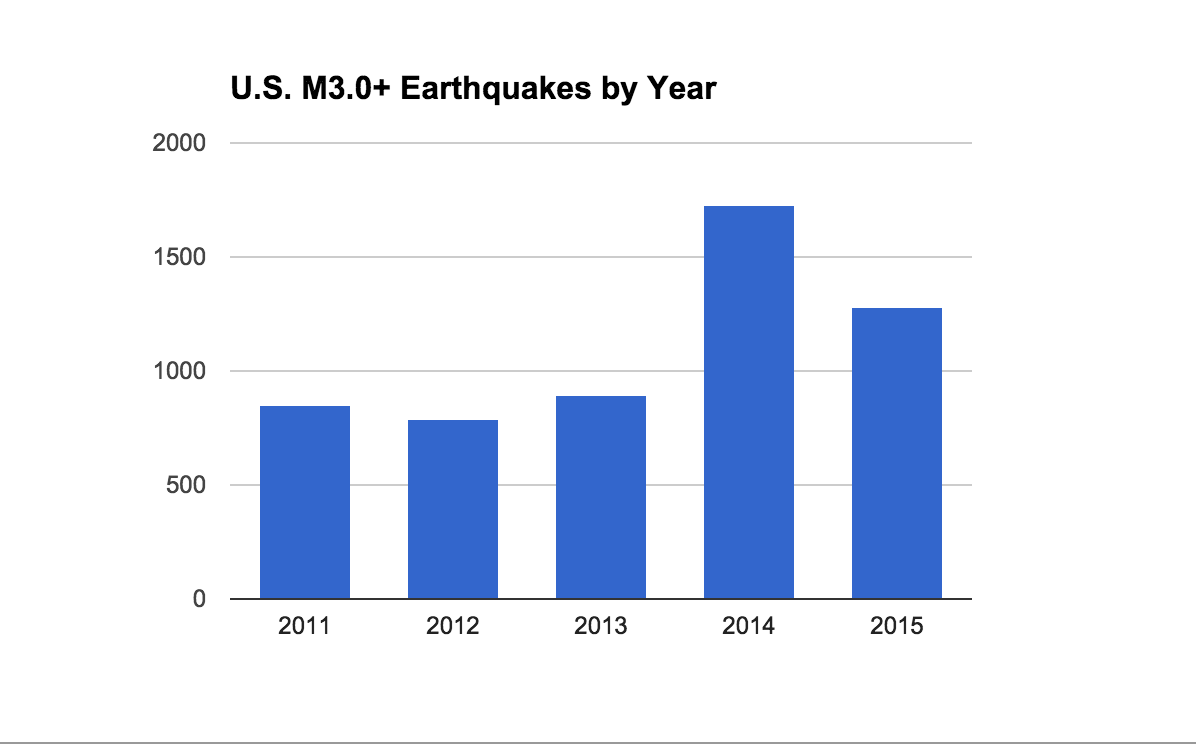So, contrary to what we could see on the map, the years 2014 and 2015 (remember that 2015 is only 3/4 done at this point) have significantly more earthquakes than the previous years. This simple bar chart ended up being pretty valuable.

### Histogram by year-month

Is it worth looking at earthquakes by year and month? Maybe. Since earthquakes aren't seasonal (we think), it's interesting to see if the number of earthquakes per month has increased over the years. For all we know, with the time-series-by-year, 2014 and 2015 each had some kind of exceptional earthquake, in which many aftershocks were triggered, but were not significantly different on a typical month-to-month basis.

How do we extract the year and month values? Take a look again at the time column:

``````    2015-09-20T16:55:21.558Z
``````

If `09` represents the month (i.e. the 9th month, i.e. September), I think you can figure out the formula using `LEFT` for yourself. Here's the resulting time series: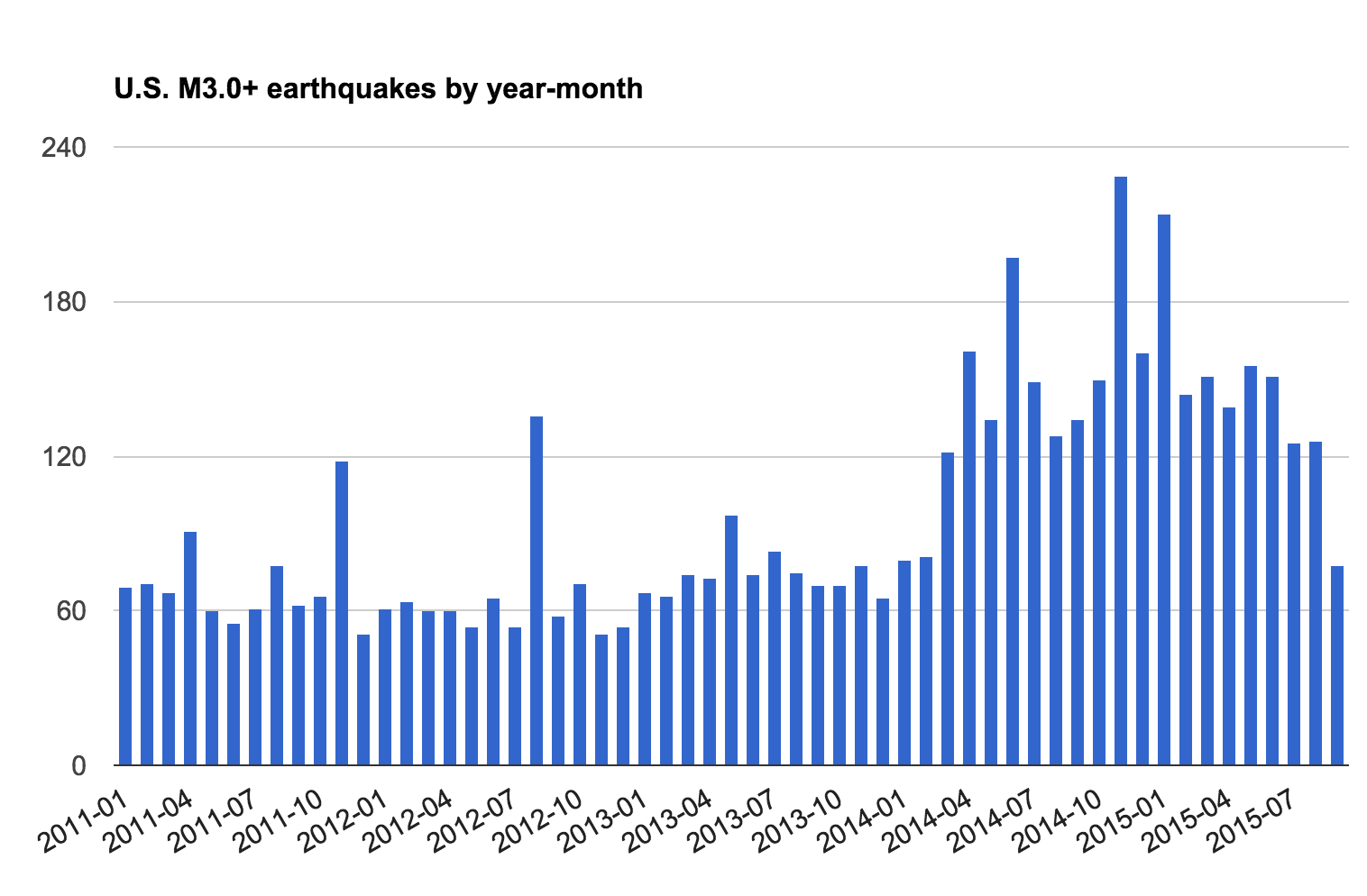At a quick glance, it does look like the overall frequency of earthquakes has markedly increased from 2014 onwards.

## The `IF` formula

Let's now go from smaller picture (i.e. earthquakes per year-month) to a broader picture: how many M3.0+ earthquakes occurred in the period of 2011 through 2013, i.e. 3 years, compared to the period of 2014 through September 2015, i.e. less than 2 years? The previous histogram makes it fairly obvious that 2014 is a new era in earthquake activity. But now we want to show, in a more black-and-white fashion, how much more dramatic the change is.

In terms of spreadsheet-steps, we basically want to create a new column that designates whether an earthquake is before 2014 or 2014 and later. We can derive this from the existing year column but we need to use a different formula.

Enter the IF function. In the example below, the formula tests cell K2 (which, in my example, is where the year column is located) to see if it is less than 2014.

If that is the case, the resulting value will be the text string, `"2011-2013"`. Else, the value will be the text string, `"2014-2015"`

``````  IF(K2 < "2014", "2011-2013", "2014-2015")
``````

Again, not to get into too much detail about formula syntax here, but the double-quotation marks are important. If you have a little knowledge of the pickiness of programming languages, you might wonder why we use the quoted-character string `"2014"` instead of the number `2014` – it's because that year column was derived from a text formula, i.e. `LEFT`.

Entering this formula and repeating it is the same as before. Your spreadsheet should look something like this (note the new era column):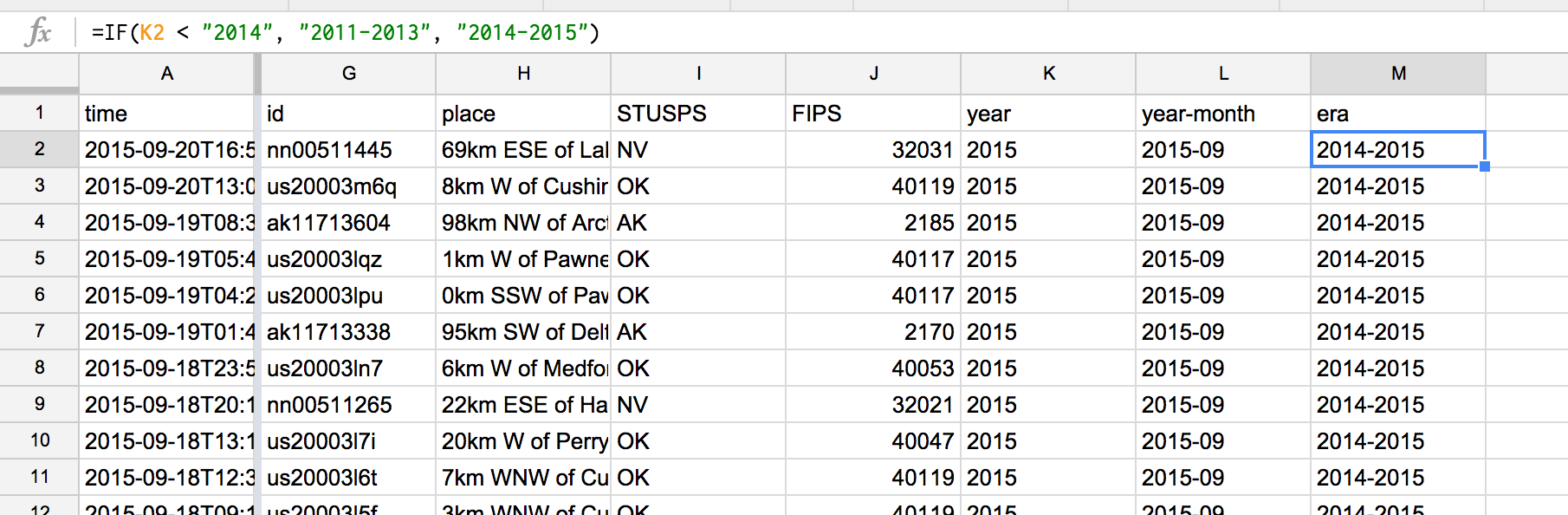### Histogram by era

What can we do with this new era column? We could create a histogram; since there are only two values – `2011-2013` and `2014-2015` – the result is a two-bar bar chart:If that's a little tame for you, go for the pie chart version:That's still not very dramatic. But think about the insight that it obscures: the blue piece of the pie represents far less time than the red piece. So the fact that it is `54.3%` of the pie is very significant. But our choice of bucket size somewhat obscures it.

Let's keep the era column for now and move on to one more kind of categorization:

### California vs. non-California

It's pretty well known that California is a big state for earthquakes. Repeat the steps we did for era, but this time, derive a column named `is_CA`, which is either `"California"` or `"Not California"` based on the value of the STUSPS column.

Assuming the STUSPS column is in column I, here's a hint to the formula:

``````=IF(I2="CA"...
``````

Then make a pie chart that looks like this: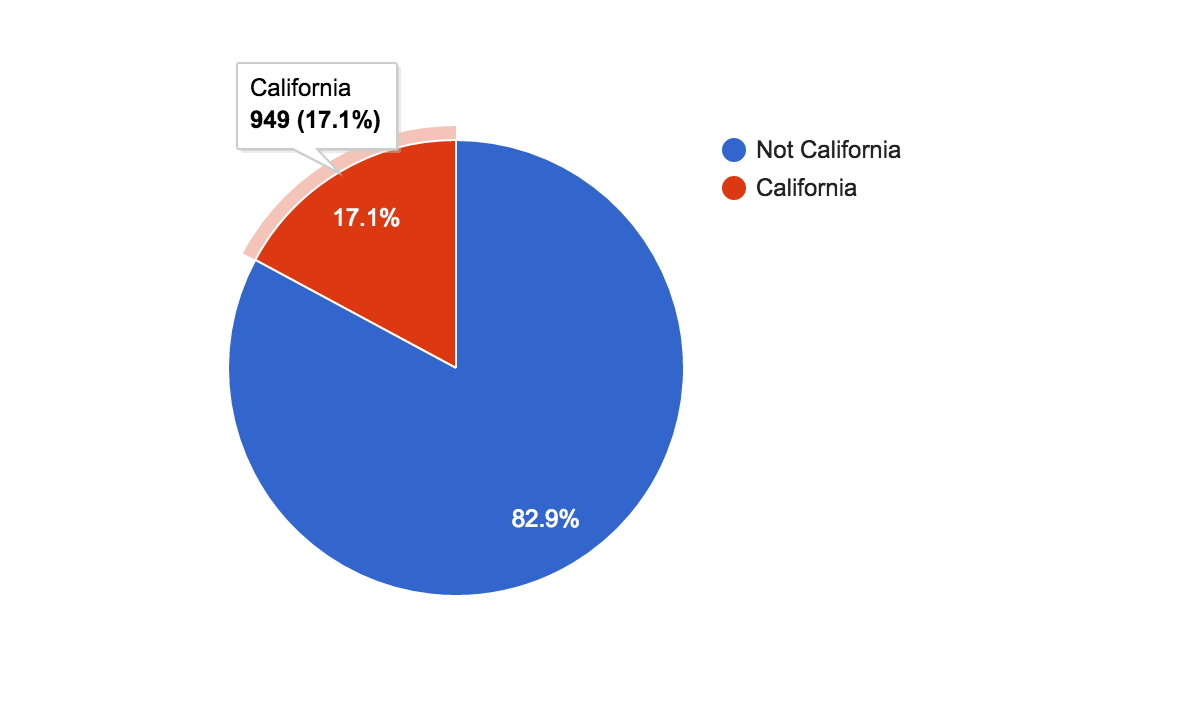## Why formulas and functions?

Again, not a terribly interesting insight. But it's good to get some practice with formulas. `LEFT` and `IF` are only a few of the possible functions we can use to transform text. But keep in mind why we need to transform text in the first place: sometimes we want to filter and sort data by categories that didn't exist in the original data, such as year.

Here is Google's searchable list of spreadsheet functions, many of which are the same in Excel. It's not worth memorizing, per se, but it's definitely worth browsing so you get an idea of what is possible.

# What we need to know

The spreadsheet techniques we've learned were fairly basic, but pretty powerful. Other than getting more familiarized with the variety of spreadsheet functions available, there's not much more to learn (at least for this class) in terms of spreadsheets, specifically.

However, there were a few things we weren't able to easily do. One of them was to just filter the earthquake data so that we could compare states with a non-trivial number of earthquakes, rather than getting something that results in this mess of a pie chart:

I use the phrase "just filter" a bit facetiously. Filtering is one of the most fundamental things we do not just as journalists, but as humans – it is our constant challenge to make sense of the world's flood of information.

Making charts from data – that is, simplifying data for visual interpretation – is one example of why and how we filter. But being able to filter data even before we visualize data is itself an essential skill.

In the next lesson, we learn about pivot tables, which are a built-in function of modern spreadsheets. In a nutshell, pivot tables allow us to easily summarize and simplify data, which we then create charts from. It doesn't sound very sexy but it is all we need for finding the most interesting insights in this earthquake data.